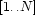#### Init Method

The `init` method is given a list of domains. Each domain is represented by the list of its values (these should be atoms or integers). A `DomainProduct` object is created as follows:

`declare O = {New DomainProduct init([D1 D2 ... Dp])}`

where each `Di` is a list of values representing a domain.

The initialization code constructs the map `value2set` by iterating through the integers in the range. For each `I` in this range, `DecodeInt` is invoked to obtain the corresponding tuple (as a list of values). For each value `V` in this tuple, `I` is added to the list of indices for `V`. When we are done, these lists of indices are turned into sets of indices.

`meth init(Domains)   Sizes = {Map Domains Length}   L1    = {Map Sizes fun {\$ Size} _#Size end}   N     = {FoldR L1 fun {\$ M#N Accu} M=Accu N*Accu end 1}   Divs  = {Map L1 fun {\$ M#_} M end}   Val2Ints = {Dictionary.new}in    for I in 1..N do       Tuple = {DecodeInt I-1 Divs Domains}   in       for V in Tuple do          {Dictionary.put Val2Ints V          I|{Dictionary.condGet Val2Ints V nil}}      end    end    divisors <- Divs   domains  <- Domains   ``    for K in {Dictionary.keys Val2Ints} do       Val2Ints.K := {FS.value.make Val2Ints.K}   end    self.range = 1#N   self.empty = FS.value.empty   self.full  = {FS.value.make self.range}   value2set <- Val2Intsend`

Denys Duchier
Version 1.2.0 (20010221)